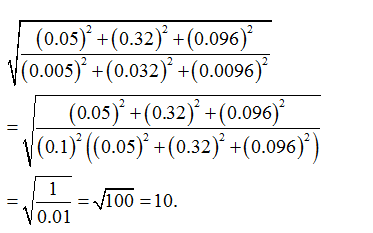• This is an assessment test.
• These tests focus on the basics of Maths and are meant to indicate your preparation level for the subject.
• Kindly take the tests in this series with a pre-defined schedule.

## Basic Maths: Test 33

Congratulations - you have completed Basic Maths: Test 33.

You scored %%SCORE%% out of %%TOTAL%%.

Your performance has been rated as %%RATING%%

 Question 1
The value of$\left( 0.25\overline{52}+0.2\overline{4} \right)$
 A 0.49 B $0.49\overline{99}$ C $0.\overline{496}$ D $0.49\overline{96}$
Question 1 Explanation:Question 2
The value of $\sqrt{\frac{{{\left( 0.05 \right)}^{2}}+{{\left( 0.32 \right)}^{2}}+{{\left( 0.096 \right)}^{2}}}{{{\left( 0.005 \right)}^{2}}+{{\left( 0.032 \right)}^{2}}+{{\left( 0.0096 \right)}^{2}}}}$
 A 0.1 B 10 C 102 D 103
Question 2 Explanation:Question 3
Simplify: $\frac{0.52\times 0.52\times 0.52+0.58\times 0.58\times 0.58}{0.52\times 0.52-0.52\times 0.58+0.58+0.58}$
 A 0.28 B 1.1 C 11 D 2.8
Question 3 Explanation:
\begin{align} & Let\,\,0.52=x\,\,and\,\,0.58=y \\ & Therefore\,\,\exp ression \\ & =\frac{\left( {{x}^{3}}+{{y}^{3}} \right)}{\left( {{x}^{2}}-xy+{{y}^{2}} \right)} \\ & =\frac{\left( x+y \right)\left( {{x}^{2}}-xy+{{y}^{2}} \right)}{\left( {{x}^{2}}-xy+{{y}^{2}} \right)} \\ & =x+y=0.52+0.58=1.10 \\ \end{align}
 Question 4
Find the value of * in the following $1\frac{5}{6}\div \frac{2}{9}\times \frac{*}{9}=2\frac{3}{4}\times \frac{5}{6}\div \frac{1}{12}$
 A $\frac{1}{30}$ B 3 C 0.3 D 30
Question 4 Explanation:
We have
\begin{align} & 1\frac{5}{6}\div \frac{2}{9}\times \frac{*}{9}=2\frac{3}{4}\times \frac{5}{6}\div \frac{1}{12} \\ & \Rightarrow \frac{11}{6}\times \frac{9}{2}\times \frac{*}{9}=\frac{11\times 5\times 12}{4\times 6} \\ & Therefore\,\,*=\frac{11\times 5\times 12\times 6\times 2\times 9}{4\times 6\times 11\times 9}=30 \\ \end{align}
 Question 5
The sum of $\sqrt{0.04}+\sqrt{0.16}+\sqrt{1.69}+\sqrt{0.0025}$ is:
 A 2.4 B 1.95 C 2.95 D 1.85
Question 5 Explanation:
\begin{align} & \sqrt{0.04}+\sqrt{0.16}+\sqrt{1.69}+\sqrt{0.0025} \\ & =0.2+0.4+1.3+0.05 \\ & =1.95 \\ \end{align}
Once you are finished, click the button below. Any items you have not completed will be marked incorrect.
There are 5 questions to complete.
 ← List →
Shaded items are complete.
 1 2 3 4 5 End